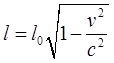## Pages

### S9. Length Contraction

First some Historical back ground.

“Length contraction was postulated by George FitzGerald (1889) and Hendrik Antoon Lorentz (1892) to explain the negative outcome of the Michelson-Morley experiment and to rescue the hypothesis of the stationary aether (Lorentz–FitzGerald contraction hypothesis). Although both FitzGerald and Lorentz alluded to the fact that electrostatic fields in motion were deformed ("Heaviside-Ellipsoid" after Oliver Heaviside, who derived this deformation from electromagnetic theory in 1888), it was considered an ad hoc hypothesis, because at this time there was no sufficient reason to assume that intermolecular forces behave the same way as electromagnetic ones.

In 1897 Joseph Larmor developed a model in which all forces are considered to be of electromagnetic origin, and length contraction appeared to be a direct consequence of this model. Yet it was shown by Henri Poincaré (1905) that electromagnetic forces alone cannot explain the electron's stability. So he had to introduce another ad hoc hypothesis: non-electric binding forces (Poincaré stresses) that ensure the electron's stability, give a dynamical explanation for length contraction, and thus hide the motion of the stationary aether.

Eventually, Albert Einstein (1905) was the first who completely removed the ad hoc character from the contraction hypothesis, by demonstrating that this contraction was no dynamical effect in the aether, but rather a kinematic effect due to the change in the notions of space, time and simultaneity brought about by special relativity. Einstein's view was further elaborated by Hermann Minkowski and others, who demonstrated the geometrical meaning of all relativistic effects in spacetime. So length contraction is ofkinematic, not dynamic, origin.” -wikipedia

Fig 1.

Suppose the system ${S}'$ moves with a uniform velocity $v$ relative to the system $S$. From the Lorentz Transformation equation we have the transformation for the end of each of the co-ordinate as referred from the origin as follows,
Now taking the difference to find the length in the two different frame, we then have;

Rearranging the expression above we have the following,

Thus,Since, $l_{0}$ is the length measured in the ${S}'$ frame and $l$ is the length measured in the stationary frame, that is the $S$ frame. We, thus conclude that since the factor in front of the $l_{0}$ is always $<1$, the length measured in the stationary frame is always less than that in the ${S}'$ frame. Or in other words, the phenomenon of length contraction is observed in the stationary frame when the length of the rod in ${S}'$ is measured.

To visualize, how the speed affects the length we can refer to figure below.

Length contraction at different speed;

The Starship Enterprise, as it approaches the speed of light.  (Velocity is given as a fraction of light speed.)  We note here, that the height of the starship does not change, and only its length that comes into play. Another important point to note is to realize how nearly imperceptible the contraction is even at 20% of light speed, and it isn't large even at 59%. Relativistic effects generally do not become large until you are very close to the speed of light. Thus, we don't feel very different even when we are moving on an jet plane or flying around a rocket capsule, that's because we only move at speed that only a tiny fraction as compared to speed of light.

This effect applies to time as well, the phenomenon of time dilation. The next note will be on the time dilation.